# PSEB 6th Class Maths Solutions Chapter 7 Algebra Ex 7.1

Punjab State Board PSEB 6th Class Maths Book Solutions Chapter 7 Algebra Ex 7.1 Textbook Exercise Questions and Answers.

## PSEB Solutions for Class 6 Maths Chapter 7 Algebra Ex 7.1

1. Find the rule which gives the number of matchsticks required to make the following ‘it’ matchstick patterns. Use a variables to write the rule:

Question (i)
A pattern of letter T asSolution:
Number of matchsticks required in a pattern of letter T = 2
Number of matchsticks required in ‘n’ patterns = 2n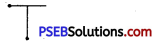Question (ii)
A pattern of letter E asSolution:
Number of matchsticks required in a pattern of letter E = 4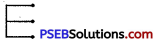Number of matchsticks required in V patterns of letter E = 4n

Question (iii)
A pattern of letter F asSolution
Number of matchsticks required in a pattern of letter F = 3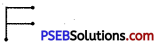Number of matchsticks required in ‘n’ patterns of letter F = 3 n

Question (iv)
A pattern of letter C asSolution:
Number of matchsticks required in a pattern of letter C = 3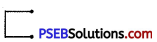Number of matchsticks required in ‘n’ patterns of letter C = 3nQuestion (v)
A pattern of letter S asSolution:
Number of matchsticks required in a pattern of letter S = 5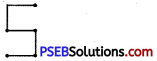Number of matchsticks required in V patterns of letter S = 5 n

2. Students are sitting in rows. There are 12 students in row. What is the rule which gives the number of students in ‘n’ rows? (Represent by table)
Solution:
Let us make a table for the number of students in ‘n’ rows.

 Number of Rows 1 2 3 4 ….. 10 …… n Number of Students 12 24 36 48 …… 120 …… 12 n

It is observed from the table that
Total number of students in ‘n’ number of rows
= (Number of Students) × (Number of rows)
= 12 × n = 12n3. The teacher distributes 3 pencils to a student What is the rule which gives the number of pencils, if there are ‘a’ number of students?
Solution:
We know
Total number of pencils
= Number of pencils × Number of students
= 3 × a = 3a

4. There are 8 pens in a pen stand. What is the rule that gives the total cost of the pens if the cost of each pen is represented by a variable ‘c’?
Solution:
We know
Total cost of the pens in ₹
= Number of pens × cost of 1 pen
= 8 × c = 8c

5. Gurleen is drawing pictures by joining dots. To make one picture,’she has to join 5 dots. Find the rule that gives the number of dots, if the number of pictures is represented by the symbol ‘p’.
Solution:
We know
Total number of dots = Number of dots × Number of pictures
= 5p6. The cost of a dozen bananas is ₹ 50. Find the rule of total cost of bananas if there are ‘d’ dozens bananas.
Solution:
We know
Total cost of bananas in ₹
= Cost of one dozen × Number of bananas
= 50 × d
= 50d

7. Look at the following matchsticks patterns of squares given below. The squares are not separate as there are two adjoined adjacent squares have a common match stick. Observe the patterns and find the rule that gives the number of matchsticks in terms of the number of squares.(Hint: If you remove the vertical stick at the end you will get a patterns of C)
Solution:

 Fig. No. No. of Squares Number of matchsticks Pattern (i) 1 4 3 x 1+ 1 (ii) 2 7 3 × 2 + 1 (iii) 3 10 3 × 3 + 1

Thus, we get the rule the number of matchsticks = 3x + 1 or 1 + 3x where x is the number of squares.